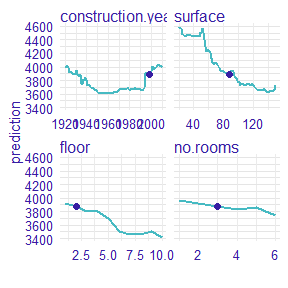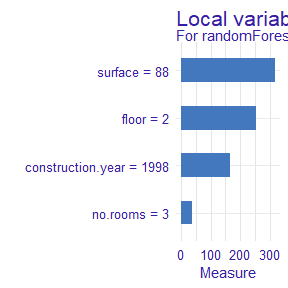# Example with random forest model

## Example with random forest regression model

In this vignette we present measure for random forest regression model.

### 1 Dataset

We work on Apartments dataset from DALEX package.

#>   m2.price construction.year surface floor no.rooms    district
#> 1     5897              1953      25     3        1 Srodmiescie
#> 2     1818              1992     143     9        5     Bielany
#> 3     3643              1937      56     1        2       Praga
#> 4     3517              1995      93     7        3      Ochota
#> 5     3013              1992     144     6        5     Mokotow
#> 6     5795              1926      61     6        2 Srodmiescie

### 2 Random forest regression model

Now, we define a random forest regression model and use explain from DALEX.

library("randomForest")
apartments_rf_model <- randomForest(m2.price ~ construction.year + surface + floor +
no.rooms, data = apartments)
explainer_rf <- explain(apartments_rf_model,
data = apartmentsTest[,2:5], y = apartmentsTest\$m2.price)
#> Preparation of a new explainer is initiated
#>   -> model label       :  randomForest  ( [33m default [39m )
#>   -> data              :  9000  rows  4  cols
#>   -> target variable   :  9000  values
#>   -> model_info        :  package randomForest , ver. 4.6.14 , task regression ( [33m default [39m )
#>   -> predict function  :  yhat.randomForest  will be used ( [33m default [39m )
#>   -> predicted values  :  numerical, min =  2096.568 , mean =  3514.335 , max =  5350.168
#>   -> residual function :  difference between y and yhat ( [33m default [39m )
#>   -> residuals         :  numerical, min =  -1328.848 , mean =  -2.811296 , max =  2164.468
#>  [32m A new explainer has been created! [39m

### 3 New observation

We need to specify an observation. Let consider a new apartment with following attributes. Moreover, we calculate predict value for this new observation.

new_apartment <- data.frame(construction.year = 1998, surface = 88, floor = 2L, no.rooms = 3)
predict(apartments_rf_model, new_apartment)
#>        1
#> 3882.104

### 4 Calculate Ceteris Paribus profiles

Let see the Ceteris Paribus Plots calculated with ceteris_paribus() function.

library("ingredients")
profiles <- ingredients::ceteris_paribus(explainer_rf, new_apartment)
plot(profiles) + show_observations(profiles)### 5 Calculate measure of local variable importance

Now, we calculated a measure of local variable importance via oscillation based on Ceteris Paribus plot. We use variant with all parameters equals to TRUE.

library("vivo")
measure <- local_variable_importance(profiles, apartments[,2:5],
absolute_deviation = TRUE, point = TRUE, density = TRUE)
plot(measure)For the new observation the most important variable is surface, then floor, construction.year and no.rooms.